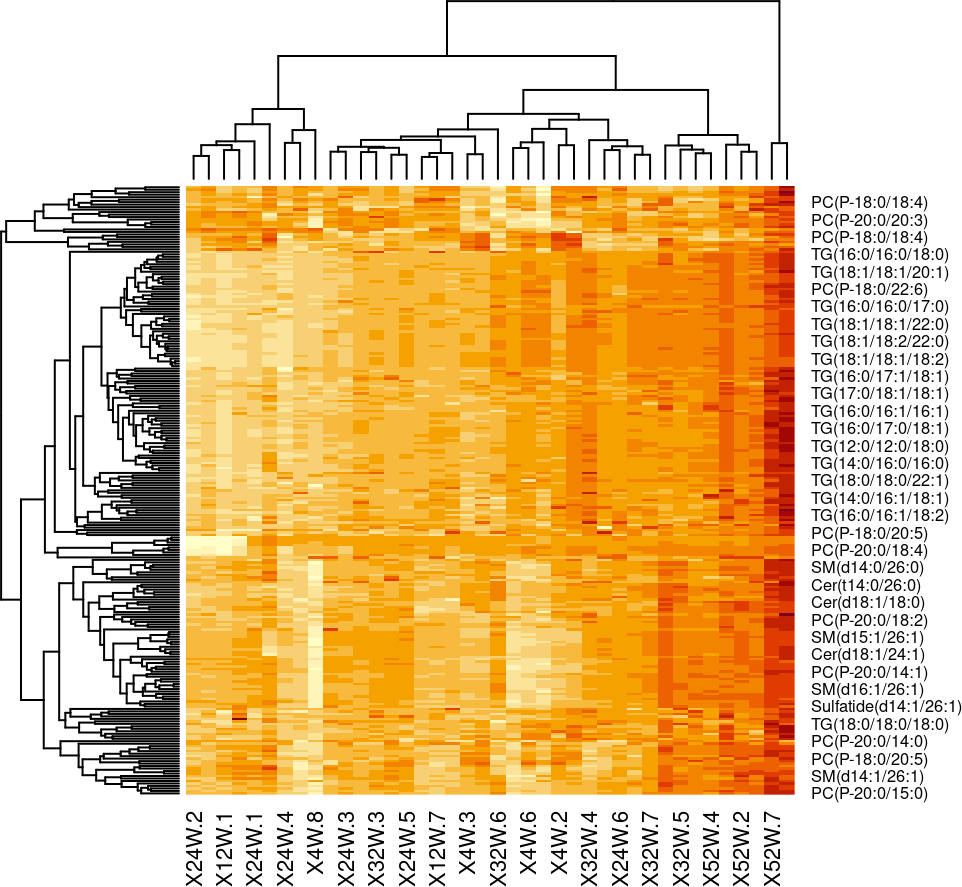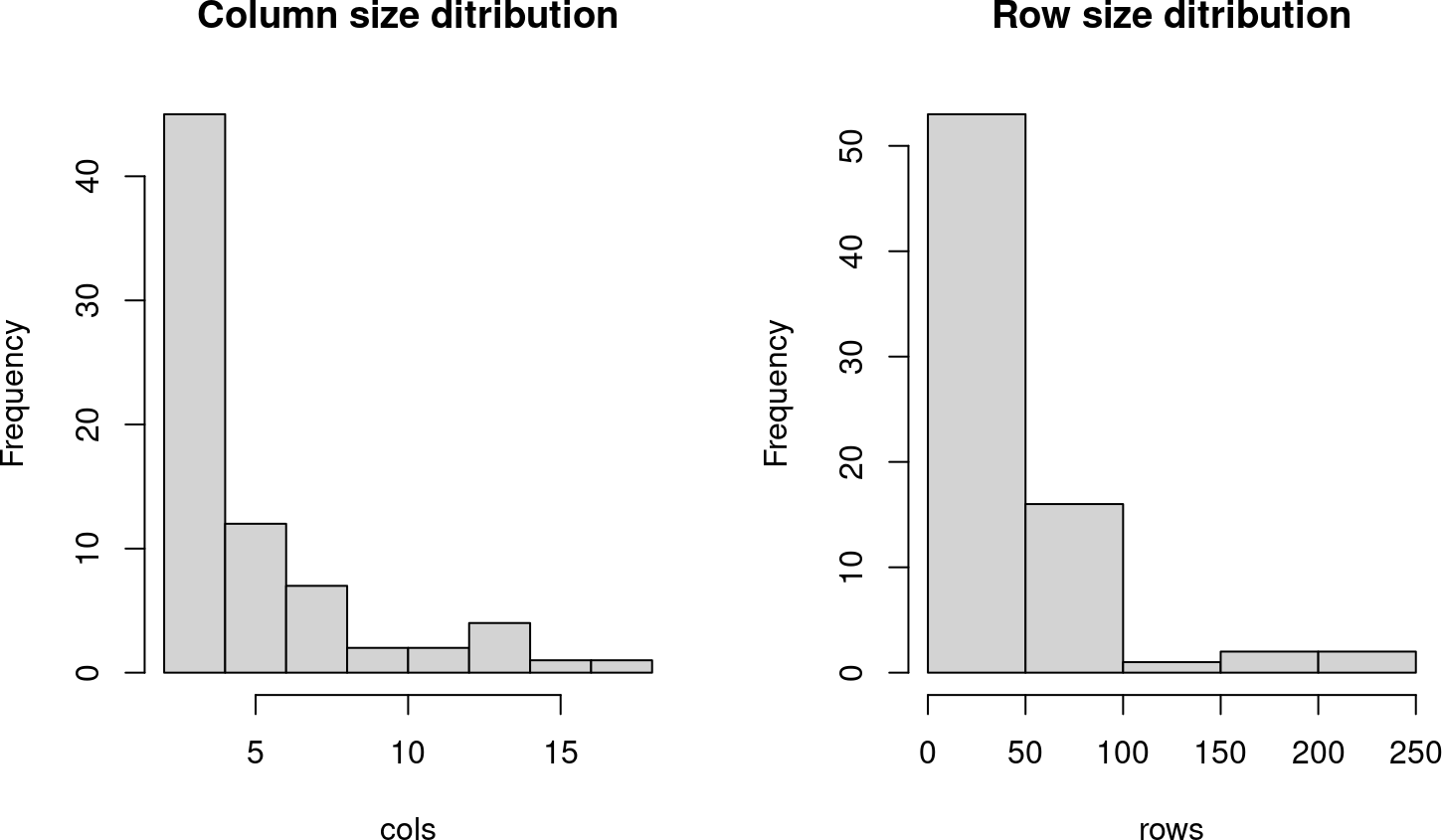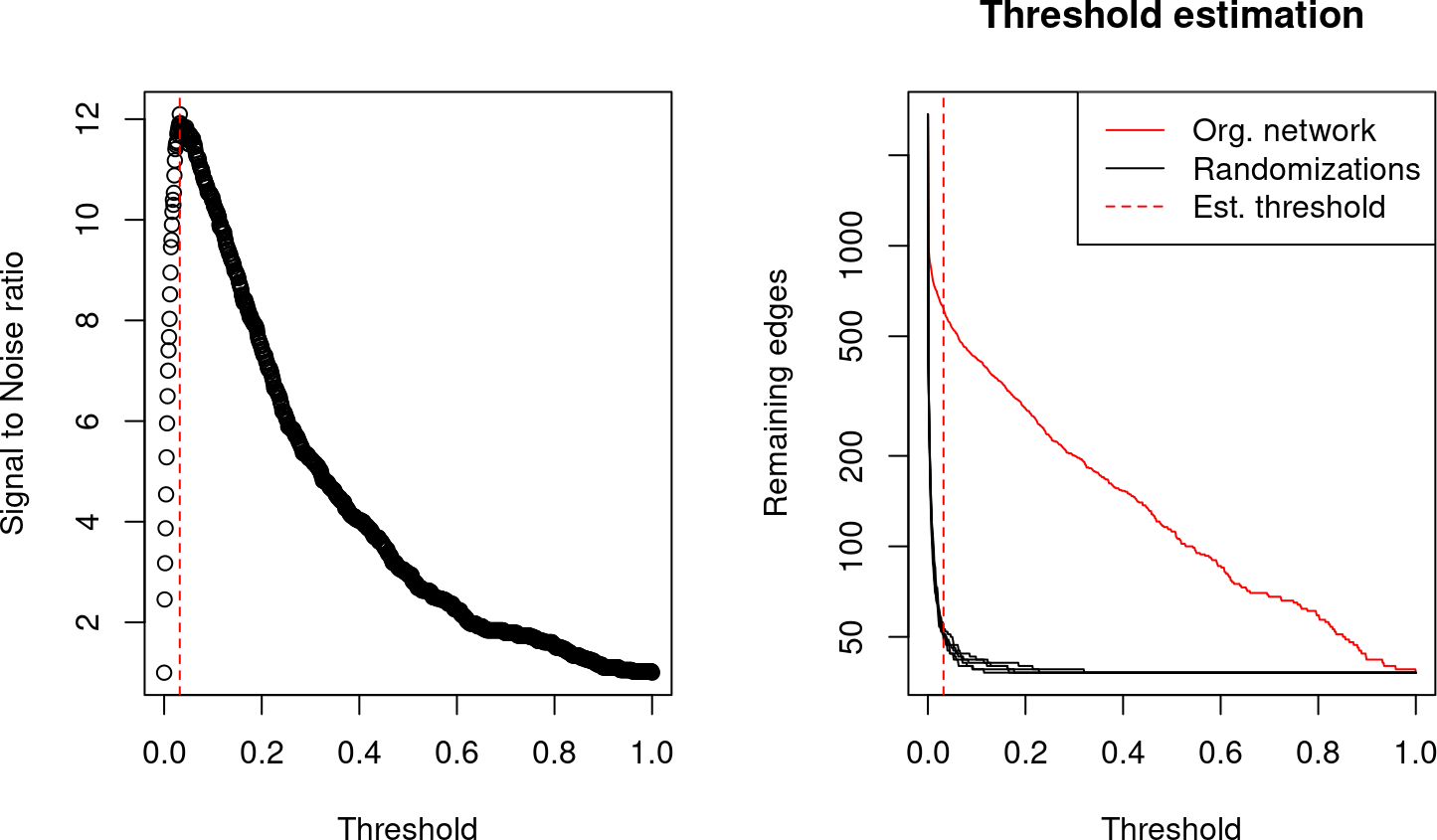# Contents

This vignette shows an example workflow for ensemble biclustering analysis with the `mosbi` package. Every function of the package has a help page with a detailed documentation. To access these type `help(package=mosbi)` in the `R` console.

Import dependencies.

``library(mosbi)``

### 0.0.2 Helper functions

Two additional functions are defined, to calculate z-scores of the data and to visualize the biclusters as a histogram.

``````z_score <- function(x, margin = 2) {
z_fun <- function(y) {
(y - mean(y, na.rm = TRUE)) / sd(y, na.rm = TRUE)
}

if (margin == 2) {
return(apply(x, margin, z_fun))
} else if (margin == 1) {
return(t(apply(x, margin, z_fun)))
}
}

bicluster_histo <- function(biclusters) {
cols <- mosbi::colhistogram(biclusters)
rows <- mosbi::rowhistogram(biclusters)

graphics::par(mfrow = c(1, 2))
hist(cols, main = "Column size ditribution")
hist(rows, main = "Row size ditribution")
}``````

Biclustering will be done on a data matrix. As an example,
lipidomics dataset from the metabolights database will be used `https://www.ebi.ac.uk/metabolights/MTBLS562`. The data consists of 40 samples (columns) and 245 lipids (rows).

``````# get data
data(mouse_data)

mouse_data <- mouse_data[c(
grep(
"metabolite_identification",
colnames(mouse_data)
),
grep("^X", colnames(mouse_data))
)]

# Make data matrix
data_matrix <- z_score(log2(as.matrix(mouse_data[2:ncol(mouse_data)])), 1)

rownames(data_matrix) <- mouse_data\$metabolite_identification

stats::heatmap(data_matrix)``````The data has a gaussian-like distribution and no missing values, so we can proceed with biclustering.

### 0.0.4 2. Compute biclusters

The `mosbi` package is able to work with results of different biclustering algorithms. The approach unites the results from different algorithms. The results of four example algorithms will be computed and converted to `mosbi::bicluster` objects. For a list of all supported biclustering algorithms/packages type `?mosbi::get_biclusters` in the `R` console.

``````# Fabia
fb <- mosbi::run_fabia(data_matrix) # In case the algorithms throws an error,
#> Cycle: 0
Cycle: 20
Cycle: 40
Cycle: 60
Cycle: 80
Cycle: 100
Cycle: 120
Cycle: 140
Cycle: 160
Cycle: 180
Cycle: 200
Cycle: 220
Cycle: 240
Cycle: 260
Cycle: 280
Cycle: 300
Cycle: 320
Cycle: 340
Cycle: 360
Cycle: 380
Cycle: 400
Cycle: 420
Cycle: 440
Cycle: 460
Cycle: 480
Cycle: 500
# return an empty list

# isa2
BCisa <- mosbi::run_isa(data_matrix)

# Plaid
BCplaid <- mosbi::run_plaid(data_matrix)
#> layer: 0
#>  5882.744
#> layer: 1
#>    0 110  19
#>   1 99 18
#>   2 94 18
#>   3 92 18
#>   4 91 18
#>  30 91 18
#>  31 18 18
#>  32 18  9
#>  33 18  9
#>  34 18  8
#>  35 18  8
#>  60 18  8
#>  5
#>  99.38514  0.00000  0.00000  0.00000
#> back fitting 2 times
#> layer: 2
#>    0 114  20
#>    1 102  17
#>   2 99 16
#>   3 98 14
#>   4 97 14
#>  30 97 14
#>  31 13 14
#>  32 13 13
#>  33 11 13
#>  34 11 10
#>  35 11 10
#>  36 11  9
#>  37 11  9
#>  60 11  9
#>  5
#>  31.94575  0.00000  0.00000  0.00000
#> back fitting 2 times
#> layer: 3
#>    0 116   9
#>    1 102   8
#>   2 98  8
#>   3 96  8
#>   4 95  8
#>  30 95  8
#>  31 55  8
#>  32 55  8
#>  33 55  8
#>  60 55  8
#>  5
#>  351.81939   0.00000   0.00000  22.67218
#> back fitting 2 times
#> layer: 4
#>    0 122  20
#>    1 101  19
#>   2 90 19
#>   3 87 19
#>   4 85 19
#>  30 85 19
#>  "Zero residual degrees of freedom"
#>  31  1 19
#>  "Zero residual degrees of freedom"
#>  32  1 19
#>  33  0 19
#>  34
#>  0 0 0 0
#>
#> Layer Rows Cols  Df      SS    MS Convergence Rows Released Cols Released
#>     0  245   40 284 6012.50 21.17          NA            NA            NA
#>     1   18    8  25  142.07  5.68           1            73            10
#>     2   11    9  19   41.04  2.16           1            86             5
#>     3   55    8  62  629.68 10.16           1            40             0

# QUBIC
BCqubic <- mosbi::run_qubic(data_matrix)

# Merge results of all algorithms
all_bics <- c(fb, BCisa, BCplaid, BCqubic)

bicluster_histo(all_bics)``````The histogram visualizes the distribution of bicluster sizes (separately for the number of rows and columns of each bicluster). The total number of found biclusters are given in the title.

### 0.0.5 3. Compute network

The next step of the ensemble approach is the computation of a similarity network of biclusters. To filter for for similarities due to random overlaps of biclusters, we apply an error model (For more details refer to our publication). Different similarity metrics are available. For details type `mosbi::bicluster_network` in the `R` console.

``````bic_net <- mosbi::bicluster_network(all_bics, # List of biclusters
data_matrix, # Data matrix
n_randomizations = 5,
# Number of randomizations for the
# error model
MARGIN = "both",
# Use datapoints for metric evaluation
metric = 4, # Fowlkes–Mallows index
# For information about the metrics,
# visit the "Similarity metrics
# evaluation" vignette
n_steps = 1000,
# At how many steps should
# the cut-of is evaluated
plot_edge_dist = TRUE
# Plot the evaluation of cut-off estimation
)
#> Esimated cut-off:  0.03203203``````The two resulting plot visualize the process of cut-off estimation. The right plot show the remaining number of edges for the computed bicluster network (red) and for randomizations of biclusters (black). The vertical red line showed the threshold with the highest signal-to-noise ratio (SNR). All evaluated SNRs are again visualized in the left plot.

The next plot shows the bicluster similarity matrix. It reveals highly similar biclusters.

``stats::heatmap(get_adjacency(bic_net))``